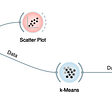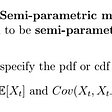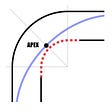# Understanding Break-even Analysis

`This post appeared in my substack newsletter on data, business and product analytics. 👉 Sign up here to get new posts straight to your inbox. 👉 Connect with me on twitter.`

One of my friends recently bought a home, and we met for dinner. He explained how that purchase will turn into a good investment in a couple of years. At some point during the discussion, he uttered a phrase, “In 18 months from now, I will hit the break-even”. I suddenly guessed he has a great analytical and business mindset and knows what he’s doing. “Break-even Analysis.”, an important analytical technique that is used by many wise investors, new businesses to know what it takes to turn things profitable. Let’s dig into this topic and understand what it is, why do you do it, and how to perform the analysis in detail.

# Definition

In simple terms, Break-even Analysis is an analytical technique to determine the least level of output (in units or dollars) the business needs to generate to fully pay off the cost of producing the goods or offering the services.

It is generally performed while developing a pricing strategy, either as part of a marketing plan or a business plan. The goal is to achieve a Break-even Point (BEP) at which the total costs and revenue are equal. That means there is no net loss or gain. Break-even.

# Why do you do it?

Say you are starting a new business and going to the bank to apply for a startup loan. One of the first questions you will face is — What’s the Break-even? The bank manager will probably not approve the loan without looking at your Break-even Analysis. She must understand how and when the startup can become profitable. The Break-even Analysis is a way to go.

Another instance where you might need to do Break-even Analysis is just before making a decision to implement a new product feature or service. This is just to understand what extra effort is required in terms of sales to generate profit. As a Product Manager, you may need to demonstrate break-even if you are presenting a new business case to your stakeholders.

# Well, How to do it?

There are 2 ways to perform Break-even Analysis. One is by estimating the number of units to sell, and another by estimating the total revenue to generate in dollars or whatever currency you are interested in. Sure, let’s dive in and check out these two methods.

# 1. Break-even Analysis in Units

Using this method, your goal is to estimate the level of output required to make the net income equal to zero. To produce output, you will have two types of costs — a Total Fixed Cost (TFC) and a Variable Cost for each unit (UVC). Variable cost increases as you sell more units. In contrast, the fixed cost remains the same. The variable cost is the added price on the actual selling price of the product. It includes costs such as commission, discounts, etc.

The formula for determining the number of units to sell to break even is derived in the following way.

`0 = n*USP - (TFC + n*UVC);  TFC = n*USP - n*UVC;  TFC = n * (USP - UVC);n = TFC / (USP - UVC);`

Where,

n = is the number of units

USP = Unit Selling Price

(TFC+n*UVC) = Total Cost

## Without Variable Cost

In an ideal case, where there is no variable cost, the number of units to sell can be simply calculated as:

`n = TFC / USP`

Note that, Total Fixed Costs should be equal to COGS plus any other expenses such as money spent on sales & marketing, research & development, and general administrative work. In this case, the Total Cost is simply the Total Fixed Cost.

What if Selling Price Varies?

Of course, the unit prices can vary depending on the sales channels use or locations you target. In those cases, you need to determine a weighted average Unit Selling Price weighing the price against % of customers for each sales channel.

For example, let’s assume you have developed an app by investing \$50,000 as a Total Fixed Cost and you have no variable cost to sell. You use different sales channels and have a weighted average Unit Selling Price of \$139 based on the above calculation. To break even, you need to sell 360 units of the app.

`n = 50,000 / 139;     = 360 (rounded up)`

On a plot, it looks like this:

The Break-even Point is the green dot on the plot.

## With Variable Cost

To account for variable cost, the UVC should be deducted from USP in the above formula as shown:

`n = TFC / (USP - UVC)`

Now, in this case, the Total Cost includes Variable Cost for each unit you sell. Therefore, the cost goes up as the number of units increase. In order to break even, you will have to sell more units or increase the USP. Let us evaluate both scenarios.

Scenario 1: Price Remains Same

Moving forward with the previous example, let’s assume that you incur a variable cost of \$35 to sell each unit. This could be the commission to the sellers or any additional cost. If so, considering the variable cost, you will have to sell 360 units of the app.

`n = 50,000 / (139-35);        = 481 (rounded up)`

On a plot, it looks like this:

Scenario 2: Number of Units to Sell Remains Same

If the price adjustment is not an issue, this exercise will help you arrive at a new USP. Let’s assume you need to maintain the number of units to sell as 360. The new USP will be.

`360 = 50,000 / (USP-35);       USP = (50,000/360)+35; USP = 174 (rounded up);`

This is simply adding the UVC to the USP for each unit.

# 2. Break-even Analysis in Dollars

The previous method is useful when you have a single product with less variability in selling prices and easy to estimate each unit’s variable cost. However, this method can be slightly complicated when you have multiple products with higher variability in costs. Also, for businesses with service offerings, this method would not fit well. To address these issues, Break-even Analysis can be done in Dollars. Let’s do that here.

To derive Break-even Point, again, the difference between the Total Revenue(TR) and combined TFC and Total Variable Cost(TVC) should be equal to the net income of zero:

`0 = TR - (TFC + TVC)`

Rearranging the formula for TR will result in:

`TR = TFC + TVC`

So, the break-even occurs when the total revenue equals the combined total fixed and variable costs.

Now, let’s look at what portion of the total revenue is available to pay off all the business’s fixed costs. This is called Contribution Rate (CR), and it is determined using the following equation.

`CR = ((TR - TVC)/TR) * 100`

Replacing TR with (TFC+TVC) in the numerator, we get:

`CR = ((TFC + TVC - TVC)/TR) * 100;    CR = (TFC/TR)* 100`

Rearranging the above formula:

`TR = (TFC/CR)* 100`

In the above equation, all you need to know is the contribution rate provided you have TFC is known. The lower we set the contribution rate, the higher the total revenue generated to get the break-even.

Going back to our example above, let’s say we set the contribution rate as 70%. That means we have 70% of the total revenue that should be available to pay off the total fixed costs. Therefore, the business needs to generate total revenue of \$71,429.

`TR = (50000/0.7)* 100;         = \$71,429 (rounded up)`

# Wrap Up

Thanks for sticking around till the end. I hope you were able to follow up on the math. The takeaway message is Break-even Analysis is an important element in every business. As I mentioned earlier, investors are keen on asking questions on break-even. It gives them a clear picture of how and when the business can start making a profit.

If you are a Product Manager, knowing how to quickly perform Break-even Analysis can help you a lot in presenting business cases to your stakeholders. You can also use this analysis in price revising exercises on existing products.

https://tinyurl.com/1r96f4c7

If you are interested in learning more about this topic, I highly recommend you to check out these resources.

1. J. Oliver; Business Math A Step-by-Step Handbook; An Open Text
2. Mike Lingle; Break even analysis made simple; Medium
3. Adam Hayes; Break-Even Analysis; Investopedia
4. Article by FreshBooks on How to Calculate the Break-Even Point
`This post appeared in my substack newsletter on data, business and product analytics. 👉 Sign up here to get new posts straight to your inbox. 👉 Connect with me on twitter.`

--

--

--

## More from Arjun Ken

Product Manager and Certified Scrum Product Owner https://arjunken.com

Love podcasts or audiobooks? Learn on the go with our new app.

## Power BI Data Sources## Diving into the Orange Tool.## A Complete Introduction To Time Series Analysis (with R):: Semi-parametric Models## Simulation of Commission Design: Part 1## Deploy your Python app with AWS Fargate — Tutorial## ARIMA for Classification with Soft Labels## Arjun Ken

Product Manager and Certified Scrum Product Owner https://arjunken.com

## What really is an insight ?## The Most Important eCommerce Metrics That You Need To Know## The Analysis Process## The Three Principles that Guide Me as a Product Leader — #1. Begin with the End in Mind